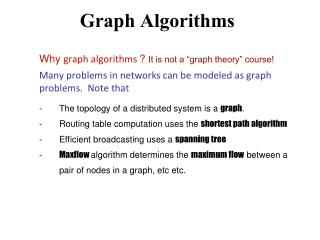# Graph Algorithms - PowerPoint PPT PresentationDownload PresentationGraph Algorithms

Graph AlgorithmsDownload Presentation## Graph Algorithms

- - - - - - - - - - - - - - - - - - - - - - - - - - - E N D - - - - - - - - - - - - - - - - - - - - - - - - - - -
##### Presentation Transcript

1. Graph Algorithms Why graph algorithms ? It is not a “graph theory” course! Many problems in networks can be modeled as graph problems. Note that - The topology of a distributed system is a graph. - Routing table computation uses the shortest path algorithm - Efficient broadcasting uses a spanning tree - Maxflow algorithm determines the maximum flow between a pair of nodes in a graph, etc etc.

2. Routing • Shortest path routing • Distance vector routing • Link state routing • Routing in sensor networks • Routing in peer-to-peer networks

3. Internet routing Autonomous System Autonomous System AS1 AS0 Each AS is under a common administration Autonomous System Autonomous System AS2 AS3 Intra-AS vs. Inter-AS routing Shortest Path First routing algorithm is the basis for OSPF

4. Most shortest path algorithms are adaptations of the classic Bellman-Ford algorithm. Works for bith undirected and directed graphs. Computes shortest path if there are no cycle of negative weight. Let D(j) = shortest distance of node j from initiator 0. D(0) = 0 (always). Initially, ∀i ≠ 0, D(i) = infinity. Routing: Shortest Path initiator w(0,m),0 0 m (w(0,j), 0 j (w(0,j)+w(j,k)), j The edge weights can represent latency or distance or some other appropriate parameter. (w(0,j)+w(j,p)), j k p Classical algorithms: Bellman-Ford, Dijkstra’s algorithm are found in most algorithm books. What is the difference between an (ordinary) graph algorithm and a distributed graph algorithm?

5. Revisiting Bellman Ford : basicidea Consider a static topology {Process 0 sends w(0,i),0 to each neighbor i} {program for process i} do message = (S,k)  S < D(i)  if parent ≠ k  parent := k fi; D(i) := S; send (D(i)+w(i,j),i) to each neighbor j ≠ parent; [] message (S,k)  S ≥ D(i) --> skip od Shortest path Current distance Computes the shortest distance to all nodes from an initiator node The parent pointers help the packets navigate to the initiator

6. Chandy & Misra’s algorithm : basic idea Consider a static topology Process 0 sends w(0,i),0 to each neighbor i {for process i > 0} do message = (S ,k)  S < D  if parent ≠ k  send ack to parent fi; parent := k; D := S; send (D + w(i,j), i) to each neighbor j ≠ parent; deficit := deficit + |N(i)| -1 [] message (S,k)  S ≥ D send ack to sender []ack  deficit := deficit – 1 [] deficit = 0  parent  i  send ack to parent od Shortest path 0 2 1 7 1 2 3 2 4 7 4 2 6 6 5 3 Combines shortest path computation with termination detection. Termination is detected when the initiator receives ack from each neighbor

7. Shortest path An important issue is: how well do such algorithms perform when the topology changes? No real network is static! Let us examine distance vector routing that is adaptation of the shortest path algorithm

8. Distance Vector D for each node i contains N elements D[i,0], D[i,1], D[i,2] … D[I, N-1]. D[i,j] is the distance from node i to node j. ∀i, D[i,i] =0, and initially ∀i,j: i≠j, D[i,j] = infinity. - Each node j periodically sends its distance vector to its immediate neighbors. - Every neighbor i of j, after receiving the broadcasts from its neighbors, updates its distance vector as follows:  k≠ i: D[i,k] = mink(w[i,j] + D[j,k] ) Used in RIP, IGRP etc Distance Vector Routing j w[i,j] D[j,k] i D[i,k] k

9. Node1 thinks d(1,3) = 2 Node 2 thinks d(2,3) = d(1,3)+1 = 3 Node 1 thinks d(1,3) = d(2,3)+1 = 4 and so on. So it will take forever for the distances to stabilize. A partial remedy is the split horizon method that will prevent 1 from sending the advertisement about d(1,3) to 2 since its first hop (to 3) is node 2 Counting to infinity Observe what can happen when the link (2,3) fails. D[j,k]=3 means j thinks k is 3 hops away  k≠ i: D[i,k] = mink(w[i,j] + D[j,k] ) Suitable for smaller networks. Larger volume of data is disseminated, but to its immediate neighbors only Poor convergence property

10. Each node i periodically broadcasts the weights of all edges (i,j) incident on it (this is the link state) to all its neighbors. The mechanism for dissemination is flooding. This helps each node eventually compute the topology of the network, and independently determine the shortest path to any destination node using some standard graph algorithm like Dijkstra’s. Link State Routing Smaller volume data disseminated over the entire network Used in OSPF of IP

11. Each link state packet has a sequence numberseq that determines the order in which the packets were generated. When a node crashes, all packets stored in it are lost. After it is repaired, new packets start with seq = 0. So these new packets may be discarded in favor of the old packets! Problem resolved using TTL Link State Routing